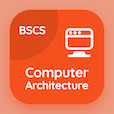Finance Online Courses (BBA)

Cost Accounting Quizzes

Cost Accounting Quiz PDF - Complete

# Process Costing System Quiz Questions Online p. 22

Learn Process Costing System quiz questions and answers, process costing system MCQ with answers PDF 22 to study Cost Accounting course online. Process Costing trivia questions, process costing system Multiple Choice Questions (MCQ) for online college degrees. "Process Costing System Quiz" PDF Book: budget indirect costs and end of financial year, fixed overhead cost variances, learning growth perspective: quality improvements, variable and fixed overhead costs, process costing system test prep for online business administration and management degree.

"The total costs incur in a production process, is divided by total number of output units, to calculate the" MCQ PDF: cost of direct labor, cost of indirect labor, cost of direct material, and unit costs for online BBA business administration. Study process costing questions and answers to improve problem solving skills for online schools for business management degrees.

## Quiz on Process Costing System MCQs

MCQ: The total costs incur in a production process, is divided by total number of output units, to calculate the

cost of indirect labor
cost of direct labor
cost of direct material
unit costs

MCQ: The process of ensuring preventive measure to be done in all machines is classified as

potential price response
potential cost response
potential budget response
potential management response

MCQ: If the number of employees who left the job are 40, the total number of employees are 200, then the employee turnover ratio will be

0.6
0.5
0.2
0.7

MCQ: If an actual cost incurred is \$627500, the flexible budget amount is \$358750, then fixed overhead variance of flexible-budget will be

\$218,750
\$238,750
\$258,750
\$268,750

MCQ: In normal costing, the situation in which the allocated amount of indirect cost is greater than the incurred cost, is considered as

incurred indirect cost
over allocated indirect cost
applied indirect cost
applied direct cost

### More Quizzes from Cost Accounting Course